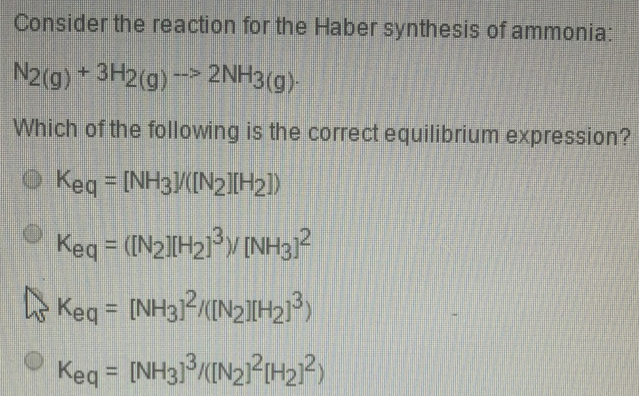Chemistry Practice Problems Equilibrium Expressions Practice Problems Solution: Consider the reaction for the Haber synthesis of a...

# Solution: Consider the reaction for the Haber synthesis of ammonia: N2(g) + 3H2 (g) → 2NH3 (g). Which of the following is the correct equilibrium expression? (i) Keq = [NH3]/[N2][H2]) (ii) Keq = ([N2][H2]3)/[NH3]2 (iii) Keq = [NH3]2/([N2][H2]3) (iv) Keq = [NH3]3/([N2]2[H2]2)

###### Problem

Consider the reaction for the Haber synthesis of ammonia:

N2(g) + 3H2 (g) → 2NH3 (g).

Which of the following is the correct equilibrium expression?

(i) Keq = [NH3]/[N2][H2])

(ii) Keq = ([N2][H2]3)/[NH3]2

(iii) Keq = [NH3]2/([N2][H2]3

(iv) Keq = [NH3]3/([N2]2[H2]2)View Complete Written Solution

Equilibrium Expressions

Equilibrium Expressions

#### Q. The equilibrium constant, Kc, for the following reaction is 2.01 x 10-2 at 710 K. NH4I (s) ⇌ NH3 (g) + HI (g) Calculate Kc at this temperature for the...

Solved • Thu Aug 30 2018 13:20:59 GMT-0400 (EDT)

Equilibrium Expressions

#### Q. Consider the following reaction N2 (g) + 3H2 (g) ⇌ 2NH3 (g) Complete the following table. Assume that all concentrations are equilibrium concentration...

Solved • Sun Aug 26 2018 07:55:04 GMT-0400 (EDT)

Equilibrium Expressions

#### Q. For A + 2B ⇌ 2C                Kc = 2.15 For 2C ⇌ D                                             Kc = 0.194 Calculate the value of the equilibrium cons...

Solved • Wed Aug 22 2018 13:36:45 GMT-0400 (EDT)

Equilibrium Expressions

#### Q. At 327 K, this reaction has a Kc values of 0.0474. X (g) + 3Y (g) ⇌ 2Z (g) Calculate Kp at 327 K. Note that the pressure is in units of atmosphere (at...

Solved • Wed Aug 22 2018 13:31:06 GMT-0400 (EDT)